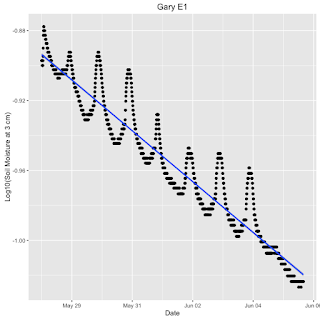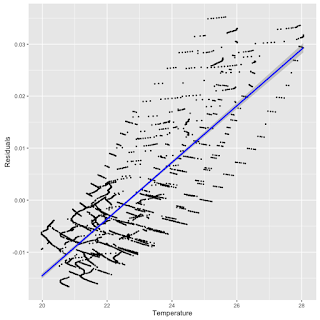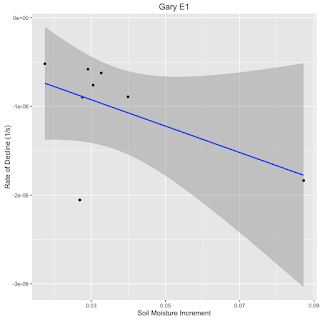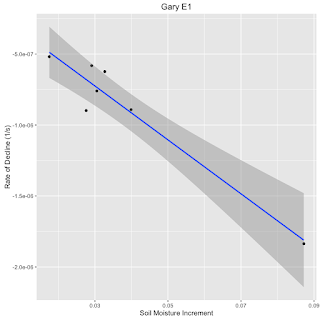## Thursday, June 23, 2016

### What processes drive soil moisture dynamics?

The primary processes driving soil moisture dynamics in the Vacant to Vibrant parcels are rainfall events that act as sources (both direct rainfall and runoff) and losses due to infiltration, evaporation, and plant mediated evapotranspiration.  In the previous blog post (here), I presented evidence that the patterns of diurnal variation in soil moisture were driven by variation in soil temperature and did not reflect the effects of the primary drivers.  The regularity of the diurnal variability and its low amplitude allows use of smoothing functions to characterizing longer term declines in soil moisture associated with the loss processes (infiltration, evaporation, and evapotranspiration).  Figure 1 shows the application a simple linear regression to a time series between rainfall events.  Using the linear fit to the time series, Figure 2 shows the that the removal of the longer-term trend emphasizes the diurnal variability, and Figure 3 shows that the variation in the residuals are correlated with observed soil temperature.Figure 1.  Semi logarithmic plot of decline of soil moisture over the period May 25 to June 6, 2016 for the Gary E1 parcel at 3 cm.  The blue line is a linear regression.Figure 2.  Plot of the residuals for the regression in Figure 1.Figure 3.  Plot of the residuals from Figure 2 showing association with measured soil temperature at 3 cm depth.  The blue line is a regression between the two variables (r= 0.80, p <0.0001).

The advantage of the linear regression in Figure 1 is that the slope is a first order (i.e. an exponential decay rate) estimate of the rate of change of soil moisture between rainfall events.  The estimated rate for the Gary E1 parcel at 3 cm soil depth is -1.68e-07 (1/s).  This method can be applied to trends at other depths or for the weighted average soil moisture and provides data for comparison of experimental and control parcels in the different soil types of the neighborhoods of the three cities in the project.

The remaining decline pattern (presented in the previous blog post) is the transient associated with a rainfall event.  Figure 4, presents the observed relation between increment of soil moisture and the subsequent first order rate of decline.Figure 4.  Relation between soil moisture increment and subsequent rate of decline in soil moisture at 3 cm depth in the Gary E1 rain garden.Figure 5.  Relation between soil moisture increment and subsequent rate of decline in soil moisture at 3 cm depth in the Gary E1 rain garden for soil moisture increments greater than 0.012.  The blue line is a regression line and the shaded area represents the standard error bounds.

The slope of the relation in Figure 5 is -1.488e-05 (r=-0.5408624, p=0.1663, NS).  The hint of an inverse relation between the increment of soil moisture following a rainfall event exists, but is not statistically significant.  The average rate of decline, however, is 1.02e-6 (1/s).    An outlier occurs on April 30, 2016.  On 4/29 and 4/30 (see Figure 6), there was a double increase.  The identification of extrema near this interval is a problem and it is reasonable to regard the April 30 point as anomalous.  Figure 7 shows the result of eliminating this point.  The estimate of the decline rate is a statistically significant -1.90e-5, which is nearly two orders of magnitude greater than the rate of decline of the inter-event interval in Figure 1.  Clearly, more data are needed to explore this relationship and its drivers, but it seems reasonable to expect that the rate of decline following a rainfall event is a function of the soil moisture gradient and the permeability of the soil.Figure 6.  Pattern of variation in soil moisture (m3/m3) at 3 cm soil depth in the Gary E1 rain garden.Figure 7.  Relation between soil moisture increment and subsequent rate of decline in soil moisture at 3 cm depth in the Gary E1 rain garden for soil moisture increments greater than 0.012 and eliminating.  The blue line is a regression line (r= -0.96, p<0.001) and the shaded area represents the standard error bounds.